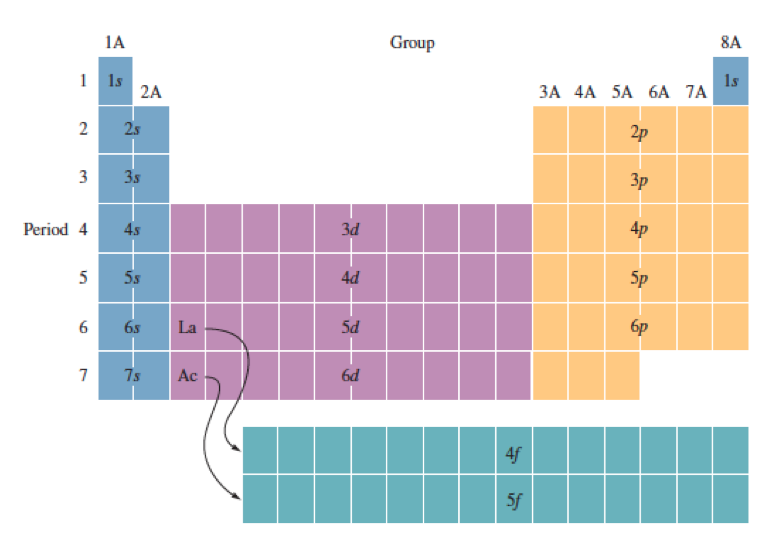# Problem: Give the ground-state electron configuration for silicon (Si).Express the complete electron configuration using superscripts where appropriate. For example,the configuration for Li would be entered as 1s22s1. Give the actual ground-stateelectron configuration for copper (Cu).Express the electron configuration using superscripts where appropriate. For example, the configuration for Li would be entered as 1s22s1.

###### FREE Expert Solution
86% (202 ratings)
###### Problem Details

Give the ground-state electron configuration for silicon (Si).

Express the complete electron configuration using superscripts where appropriate. For example,the configuration for Li would be entered as 1s22s1.

Give the actual ground-stateelectron configuration for copper (Cu).

Express the electron configuration using superscripts where appropriate. For example, the configuration for Li would be entered as 1s22s1.What scientific concept do you need to know in order to solve this problem?

Our tutors have indicated that to solve this problem you will need to apply the The Electron Configurations: Exceptions concept. You can view video lessons to learn The Electron Configurations: Exceptions. Or if you need more The Electron Configurations: Exceptions practice, you can also practice The Electron Configurations: Exceptions practice problems.

What is the difficulty of this problem?

Our tutors rated the difficulty ofGive the ground-state electron configuration for silicon (Si...as medium difficulty.

How long does this problem take to solve?

Our expert Chemistry tutor, Jules took 3 minutes and 15 seconds to solve this problem. You can follow their steps in the video explanation above.

What professor is this problem relevant for?

Based on our data, we think this problem is relevant for Professor Porter's class at IUPUI.